# How to Convert Numbers to Year/Month/Day or Date in Excel?

Have you ever tried to convert the number of days to years, months, and days in Excel? If we try to do this by hand, it can be a time-consuming process because we need to divide to get the year, age it to get the months, and then repeat the process to get the days. We can solve this process in a more straightforward and time-efficient manner. Read this tutorial to learn how you can convert numbers to years, months, and days in Excel.

## Converting Numbers to Year/Month/Day in Excel

Here we will use the DATEDIF formula to get any one of the results, then use the auto-fill handle to get all the results. Let's go over a simple procedure for converting numbers to years, months, and days in Excel using formulas.

### Step 1

Consider the following image of an excel sheet with a list of numbers.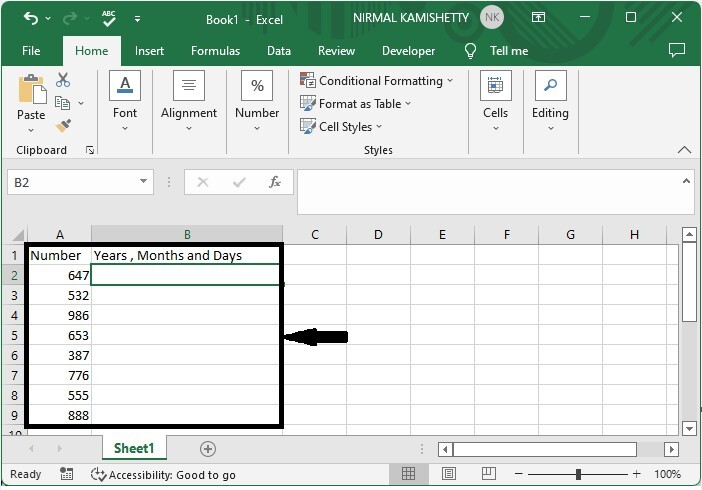Now, in our case, cell B2, click on an empty cell and enter the following formula −

=DATEDIF(0,A2,"y"), "years"; +DATEDIF(0,A2,"ym"), "months"; +DATEDIF(0,A2,"md"), "days" and press enter to get our first result. In the formula, A2 is the address of our value in the Excel sheet.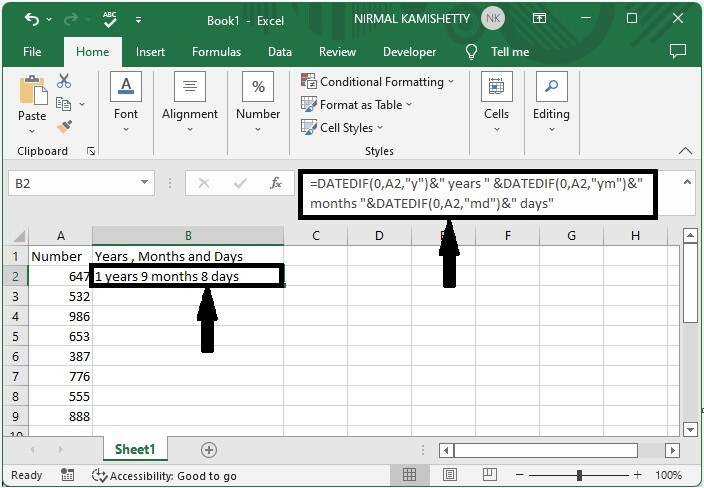### Step 2

Now drag from the first result using the auto-fill handle to get all the results and our final output will be similar to the below image.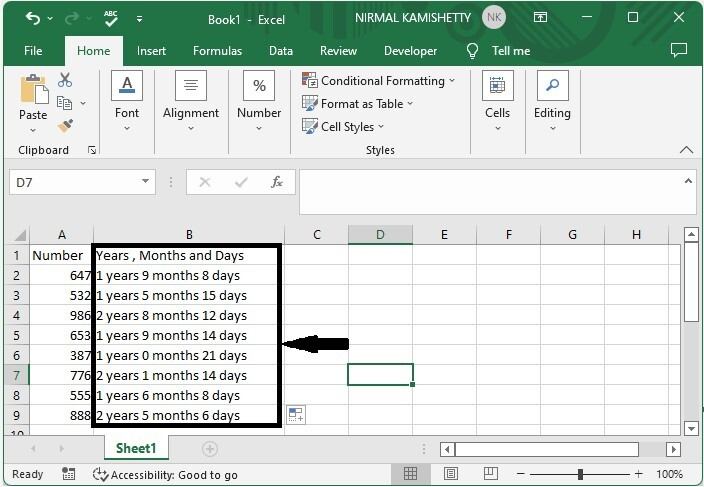## Converting Numbers to Date in Excel

Here we will use the short date function to complete our task. Let's go over a simple procedure for converting numbers to dates in Excel.

### Step 1

Let us consider the same data that we used in the above example. Now, to convert the numbers to dates, select the date, then click on the number and select the short date from the list.

Select date > Home > Number > Short date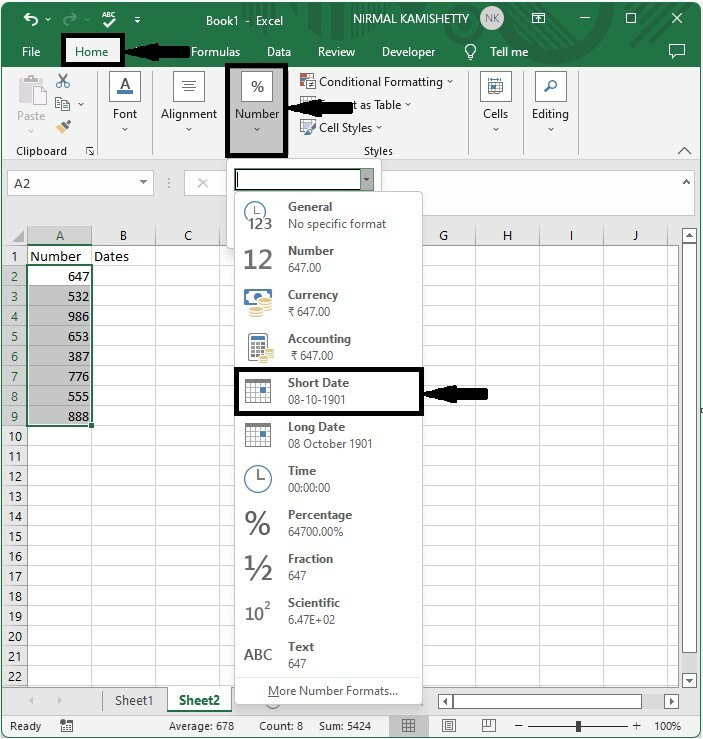And the end result will be similar to the image below.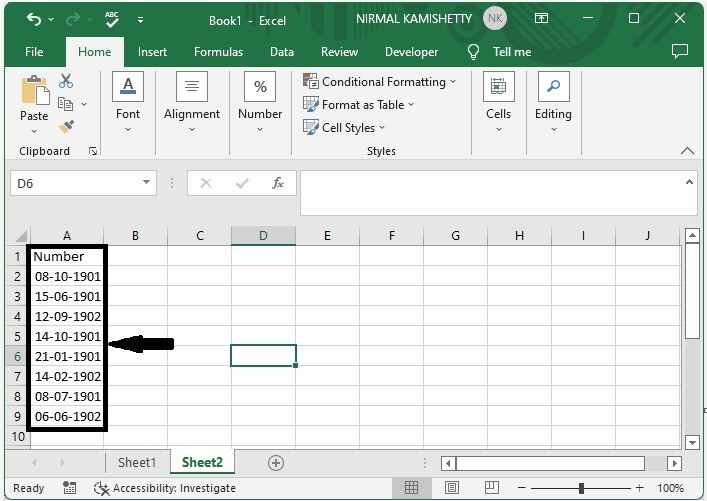## Conclusion

In this tutorial, we used a simple example to demonstrate how you can convert numbers to data in Excel.